• Slides: 94Topic 9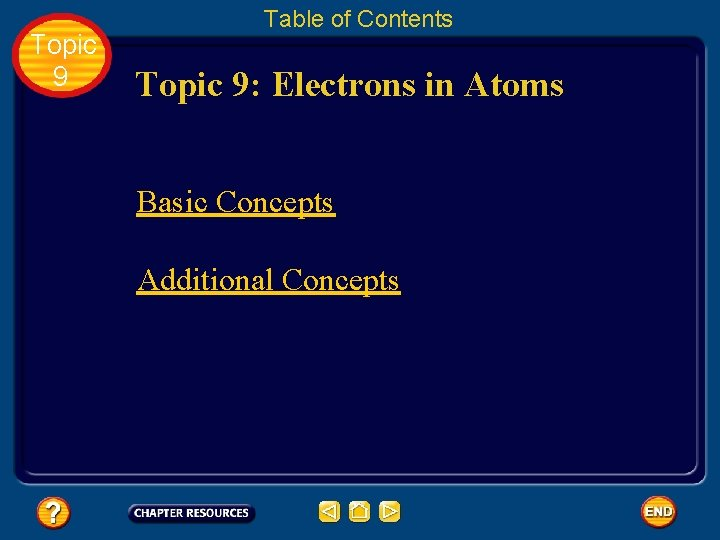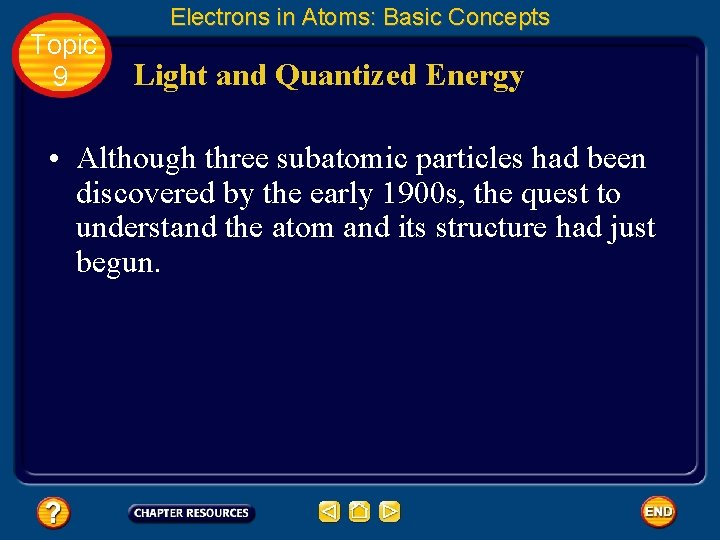Topic 9 Electrons in Atoms: Basic Concepts Light and Quantized Energy • Although three subatomic particles had been discovered by the early 1900 s, the quest to understand the atom and its structure had just begun.Topic 9 Electrons in Atoms: Basic Concepts Light and Quantized Energy • Rutherford proposed that all of an atom’s positive charge and virtually all of its mass are concentrated in a nucleus that is surrounded by fast-moving electrons. • Although his nuclear model was a major scientific development, it lacked detail about how electrons occupy the space surrounding the nucleus.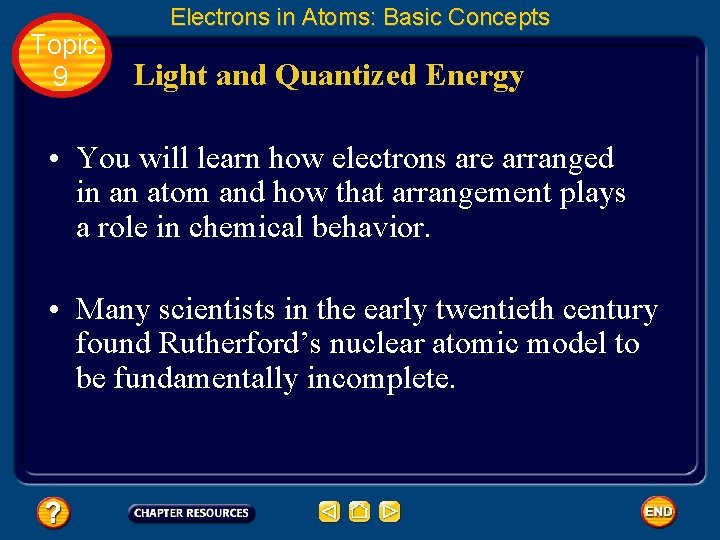Topic 9 Electrons in Atoms: Basic Concepts Light and Quantized Energy • You will learn how electrons are arranged in an atom and how that arrangement plays a role in chemical behavior. • Many scientists in the early twentieth century found Rutherford’s nuclear atomic model to be fundamentally incomplete.Topic 9 Electrons in Atoms: Basic Concepts Light and Quantized Energy • To physicists, the model did not explain how the atom’s electrons are arranged in the space around the nucleus. • Nor did it address the question of why the negatively charged electrons are not pulled into the atom’s positively charged nucleus.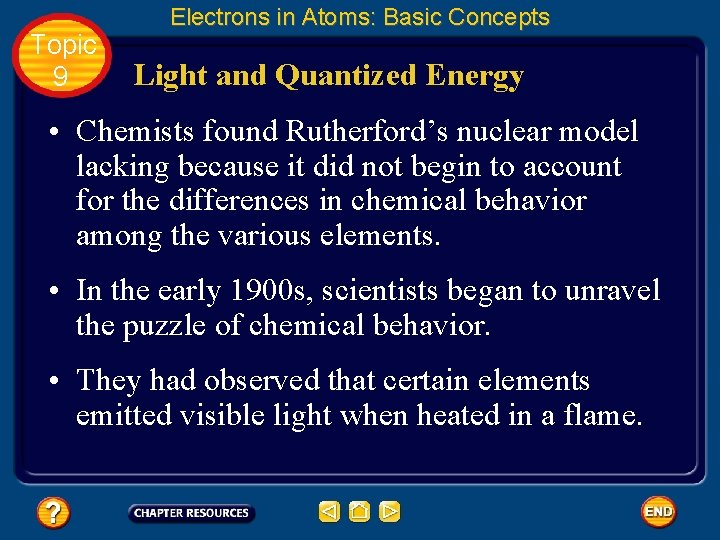Topic 9 Electrons in Atoms: Basic Concepts Light and Quantized Energy • Chemists found Rutherford’s nuclear model lacking because it did not begin to account for the differences in chemical behavior among the various elements. • In the early 1900 s, scientists began to unravel the puzzle of chemical behavior. • They had observed that certain elements emitted visible light when heated in a flame.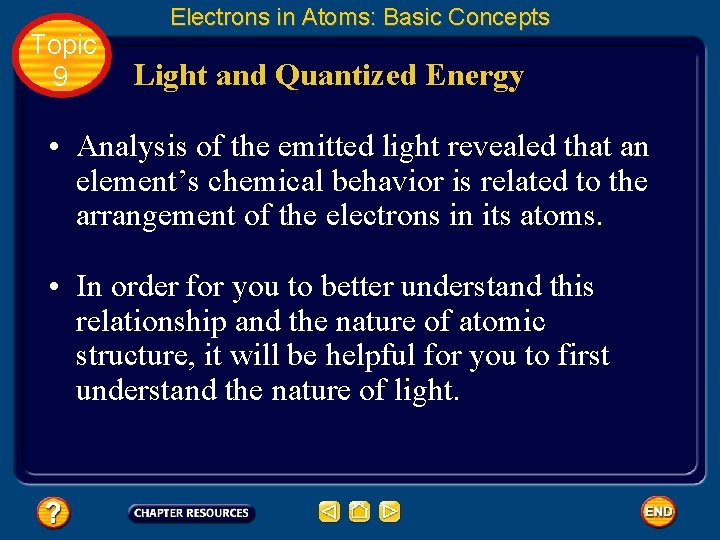Topic 9 Electrons in Atoms: Basic Concepts Light and Quantized Energy • Analysis of the emitted light revealed that an element’s chemical behavior is related to the arrangement of the electrons in its atoms. • In order for you to better understand this relationship and the nature of atomic structure, it will be helpful for you to first understand the nature of light.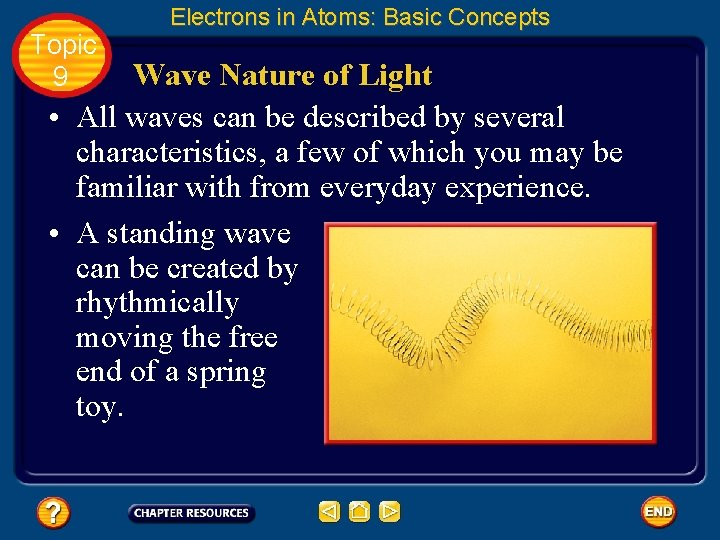Topic 9 Electrons in Atoms: Basic Concepts Wave Nature of Light • All waves can be described by several characteristics, a few of which you may be familiar with from everyday experience. • A standing wave can be created by rhythmically moving the free end of a spring toy.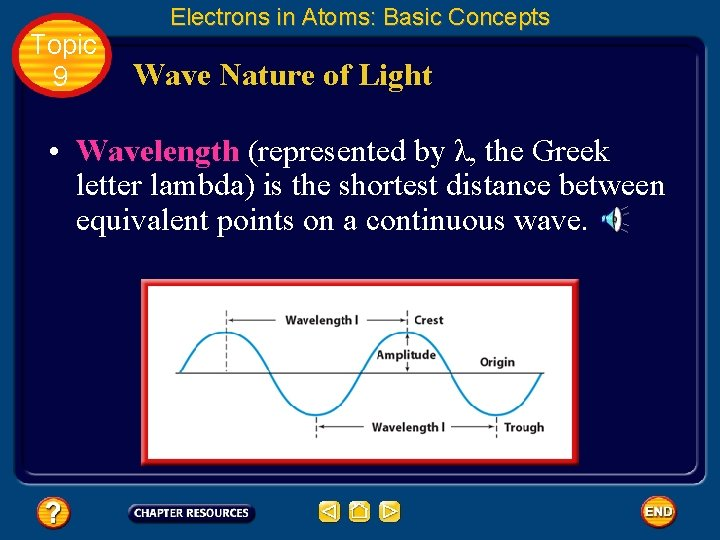Topic 9 Electrons in Atoms: Basic Concepts Wave Nature of Light • Wavelength (represented by λ, the Greek letter lambda) is the shortest distance between equivalent points on a continuous wave.Topic 9 Electrons in Atoms: Basic Concepts Wave Nature of Light • The wavelength is measured from crest to crest or from trough to trough. • Wavelength is usually expressed in meters, centimeters, or nanometers (1 nm = 1 x 10– 9 m).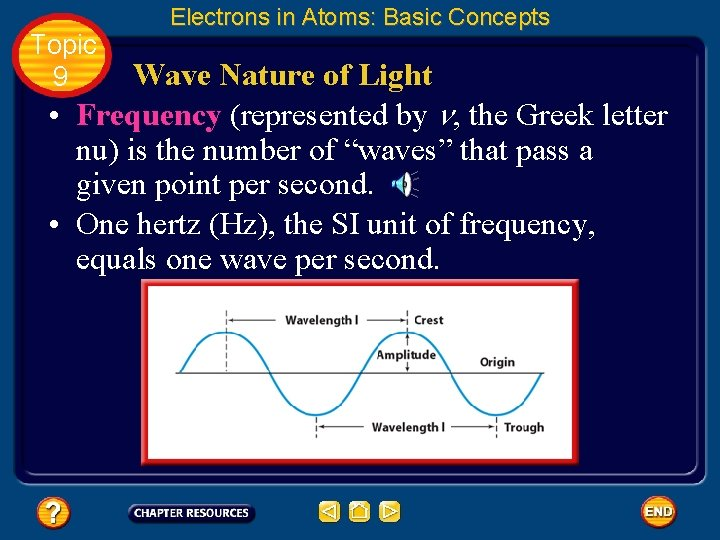Topic 9 Electrons in Atoms: Basic Concepts Wave Nature of Light • Frequency (represented by n, the Greek letter nu) is the number of “waves” that pass a given point per second. • One hertz (Hz), the SI unit of frequency, equals one wave per second.Topic 9 Electrons in Atoms: Basic Concepts Wave Nature of Light • In calculations, frequency is expressed with units of “waves per second, ” ( ) or (s– 1) where the term “waves” is understood.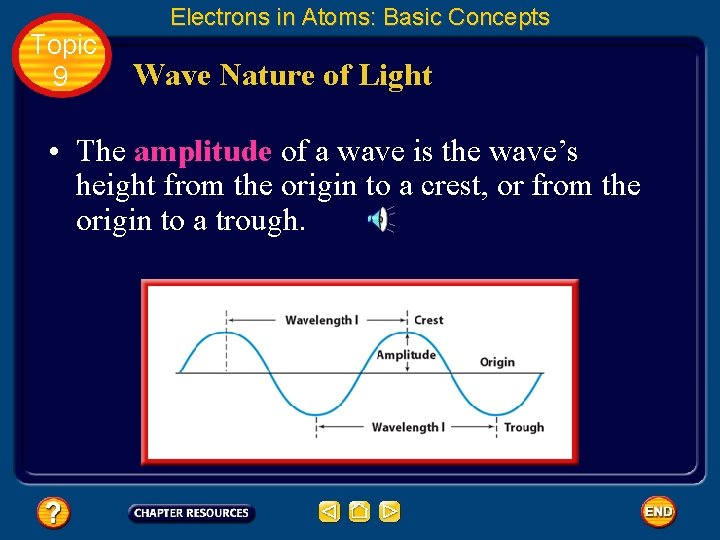Topic 9 Electrons in Atoms: Basic Concepts Wave Nature of Light • The amplitude of a wave is the wave’s height from the origin to a crest, or from the origin to a trough.Topic 9 Electrons in Atoms: Basic Concepts Wave Nature of Light • All electromagnetic waves, including visible light, travel at a speed of 3. 00 x 108 m/s in a vacuum. • Because the speed of light is such an important and universal value, it is given its own symbol, c. • The speed of light is the product of its wavelength (λ) and its frequency (n).Topic 9 Electrons in Atoms: Basic Concepts Wave Nature of Light • Although the speed of all electromagnetic waves is the same, waves may have different wavelengths and frequencies. • As you can see from the equation, wavelength and frequency are inversely related; in other words, as one quantity increases, the other decreases.Topic 9 Electrons in Atoms: Basic Concepts Wave Nature of Light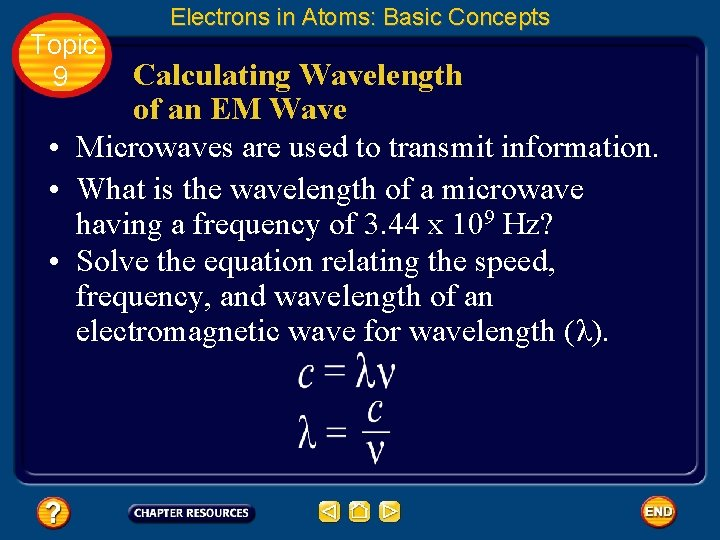Topic 9 Electrons in Atoms: Basic Concepts Calculating Wavelength of an EM Wave • Microwaves are used to transmit information. • What is the wavelength of a microwave having a frequency of 3. 44 x 109 Hz? • Solve the equation relating the speed, frequency, and wavelength of an electromagnetic wave for wavelength (λ).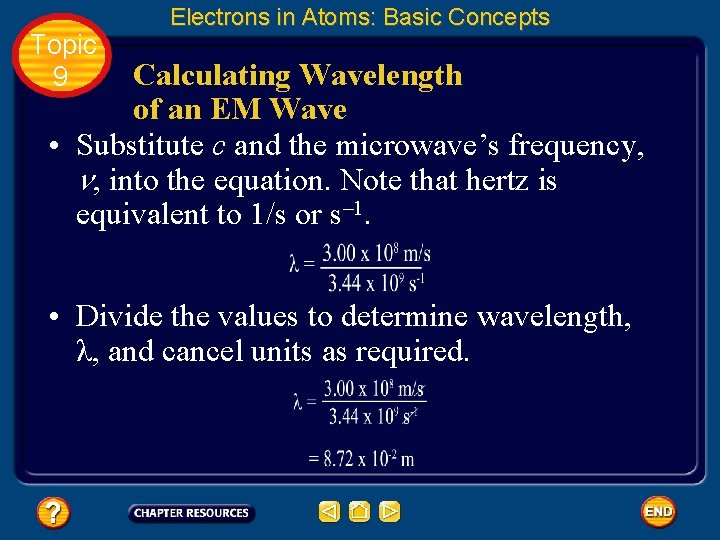Topic 9 Electrons in Atoms: Basic Concepts Calculating Wavelength of an EM Wave • Substitute c and the microwave’s frequency, n, into the equation. Note that hertz is equivalent to 1/s or s– 1. • Divide the values to determine wavelength, λ, and cancel units as required.Topic 9 Electrons in Atoms: Basic Concepts Particle Nature of Light • While considering light as a wave does explain much of its everyday behavior, it fails to adequately describe important aspects of light’s interactions with matter.Topic 9 Electrons in Atoms: Basic Concepts Particle Nature of Light • The wave model of light cannot explain why heated objects emit only certain frequencies of light at a given temperature, or why some metals emit electrons when colored light of a specific frequency shines on them. • Obviously, a totally new model or a revision of the current model of light was needed to address these phenomena.Topic 9 Electrons in Atoms: Basic Concepts The quantum concept • In 1900, the German physicist Max Planck (1858– 1947) began searching for an explanation as he studied the light emitted from heated objects. Click box to view movie clip.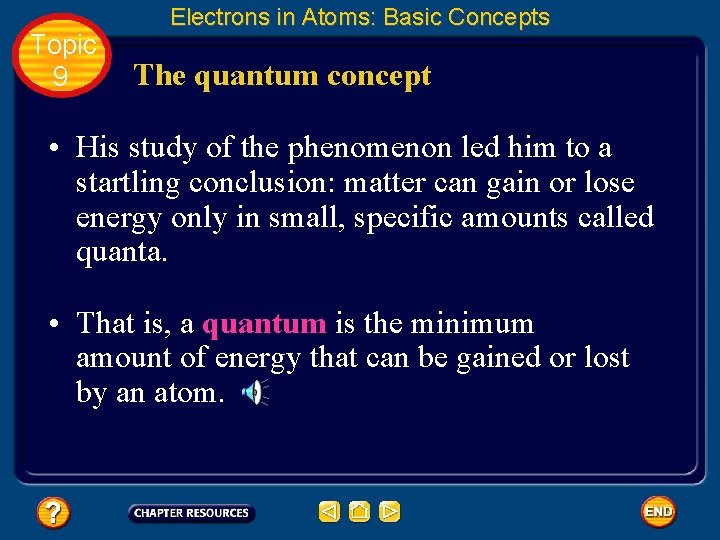Topic 9 Electrons in Atoms: Basic Concepts The quantum concept • His study of the phenomenon led him to a startling conclusion: matter can gain or lose energy only in small, specific amounts called quanta. • That is, a quantum is the minimum amount of energy that can be gained or lost by an atom.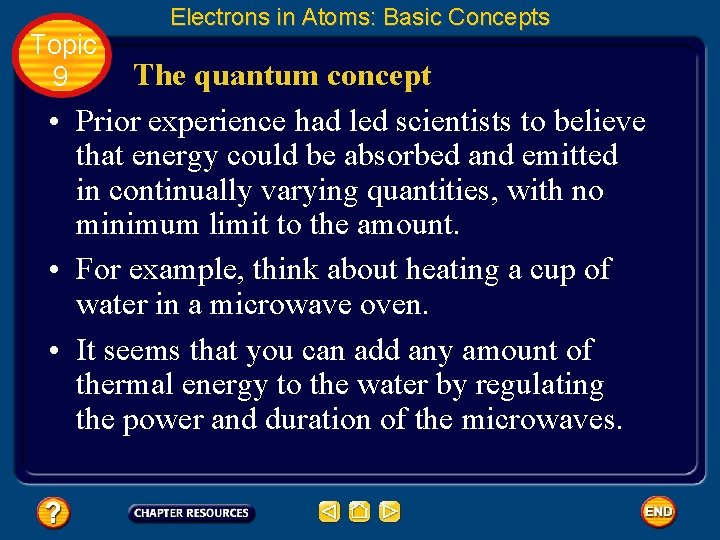Topic 9 Electrons in Atoms: Basic Concepts The quantum concept • Prior experience had led scientists to believe that energy could be absorbed and emitted in continually varying quantities, with no minimum limit to the amount. • For example, think about heating a cup of water in a microwave oven. • It seems that you can add any amount of thermal energy to the water by regulating the power and duration of the microwaves.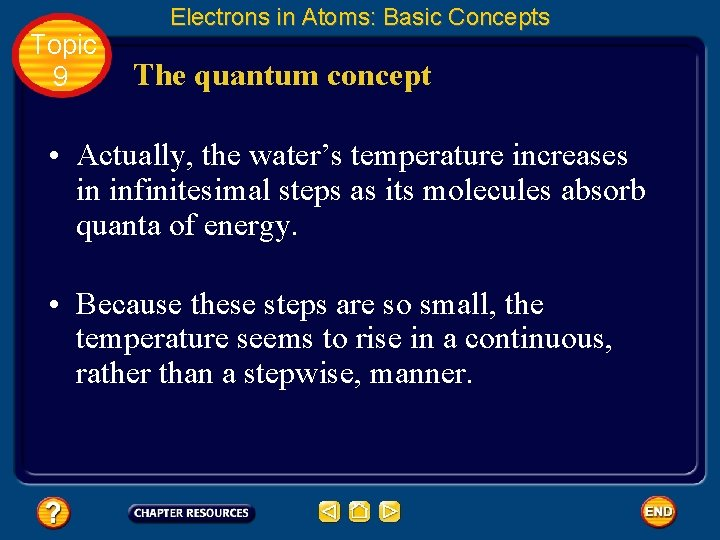Topic 9 Electrons in Atoms: Basic Concepts The quantum concept • Actually, the water’s temperature increases in infinitesimal steps as its molecules absorb quanta of energy. • Because these steps are so small, the temperature seems to rise in a continuous, rather than a stepwise, manner.Topic 9 Electrons in Atoms: Basic Concepts The quantum concept • He then went further and demonstrated mathematically that the energy of a quantum is related to the frequency of the emitted radiation by the equation where E is energy, h is Planck’s constant, and v is frequency.Topic 9 Electrons in Atoms: Basic Concepts The quantum concept • Planck’s constant has a value of 6. 626 x 10– 34 J · s, where J is the symbol for the joule, the SI unit of energy. • Looking at the equation, you can see that the energy of radiation increases as the radiation’s frequency, v, increases.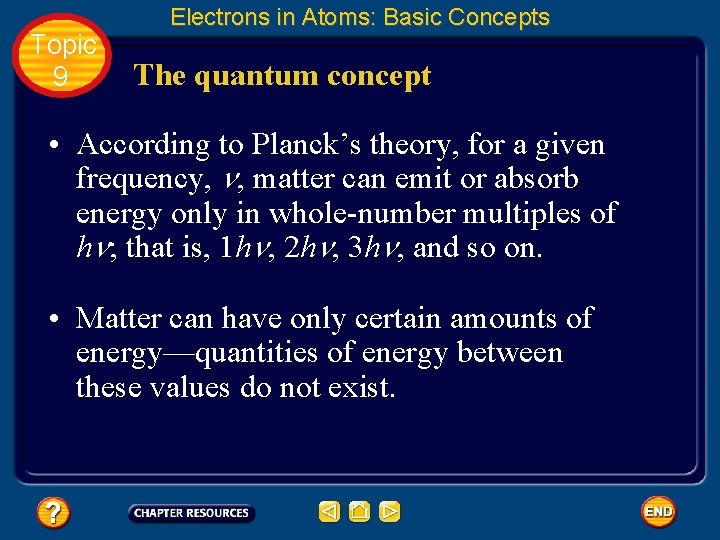Topic 9 Electrons in Atoms: Basic Concepts The quantum concept • According to Planck’s theory, for a given frequency, n, matter can emit or absorb energy only in whole-number multiples of hn; that is, 1 hn, 2 hn, 3 hn, and so on. • Matter can have only certain amounts of energy—quantities of energy between these values do not exist.Topic 9 Electrons in Atoms: Basic Concepts The photoelectric effect • Scientists knew that the wave model (still very popular in spite of Planck’s proposal) could not explain a phenomenon called the photoelectric effect.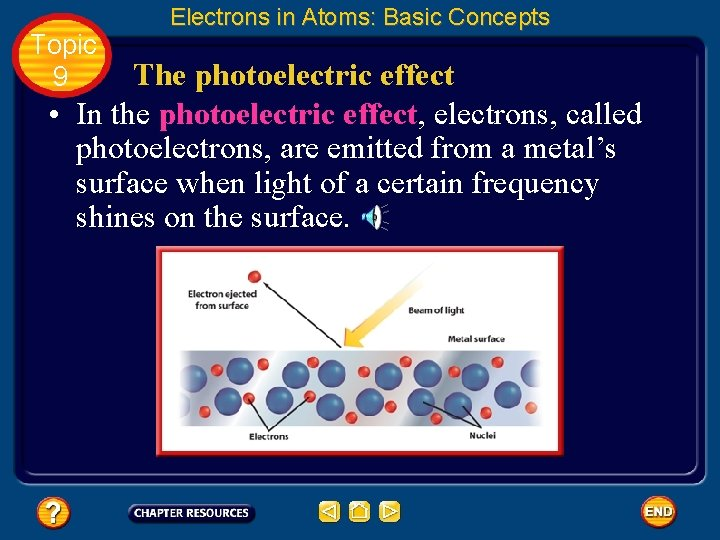Topic 9 Electrons in Atoms: Basic Concepts The photoelectric effect • In the photoelectric effect, electrons, called photoelectrons, are emitted from a metal’s surface when light of a certain frequency shines on the surface.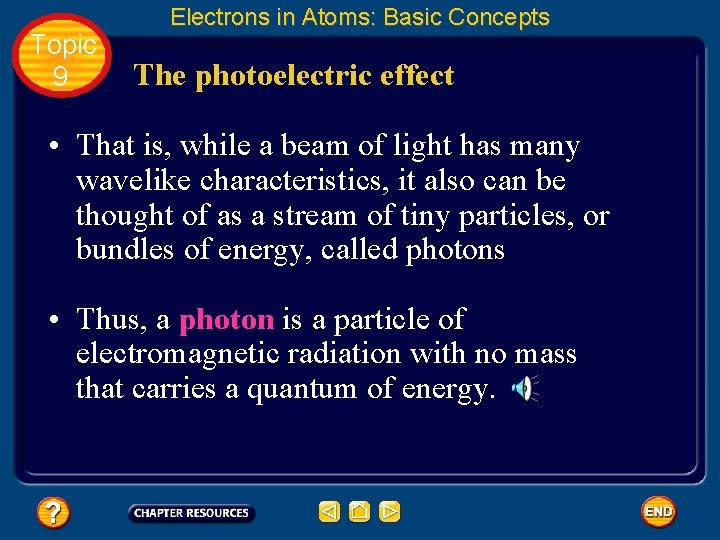Topic 9 Electrons in Atoms: Basic Concepts The photoelectric effect • That is, while a beam of light has many wavelike characteristics, it also can be thought of as a stream of tiny particles, or bundles of energy, called photons • Thus, a photon is a particle of electromagnetic radiation with no mass that carries a quantum of energy.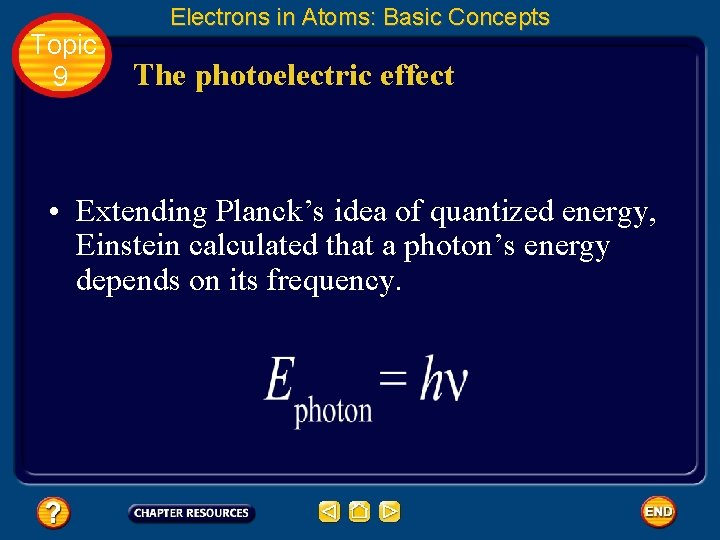Topic 9 Electrons in Atoms: Basic Concepts The photoelectric effect • Extending Planck’s idea of quantized energy, Einstein calculated that a photon’s energy depends on its frequency.Topic 9 Electrons in Atoms: Basic Concepts The photoelectric effect • Further, Einstein proposed that the energy of a photon of light must have a certain minimum, or threshold, value to cause the ejection of a photoelectron. • That is, for the photoelectric effect to occur, a photon must possess, at a minimum, the energy required to free an electron from an atom of the metal.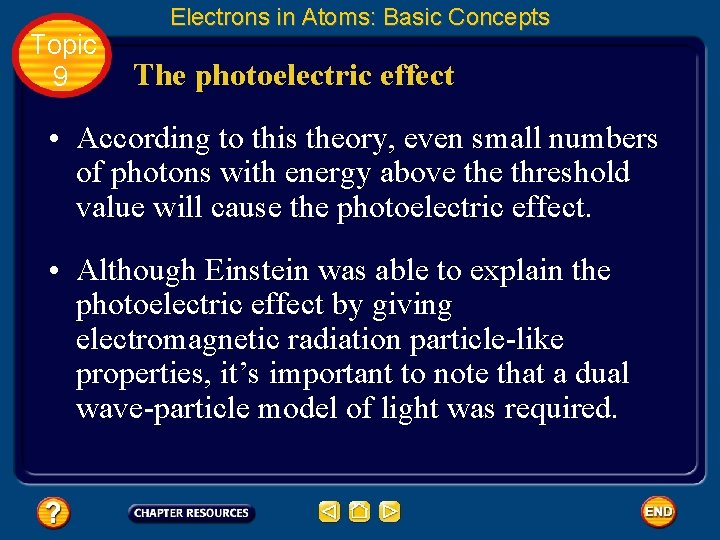Topic 9 Electrons in Atoms: Basic Concepts The photoelectric effect • According to this theory, even small numbers of photons with energy above threshold value will cause the photoelectric effect. • Although Einstein was able to explain the photoelectric effect by giving electromagnetic radiation particle-like properties, it’s important to note that a dual wave-particle model of light was required.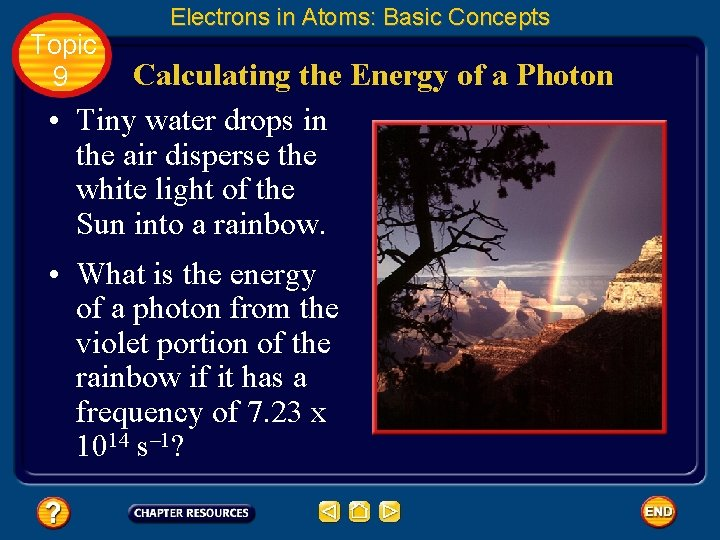Topic 9 Electrons in Atoms: Basic Concepts Calculating the Energy of a Photon • Tiny water drops in the air disperse the white light of the Sun into a rainbow. • What is the energy of a photon from the violet portion of the rainbow if it has a frequency of 7. 23 x 1014 s– 1?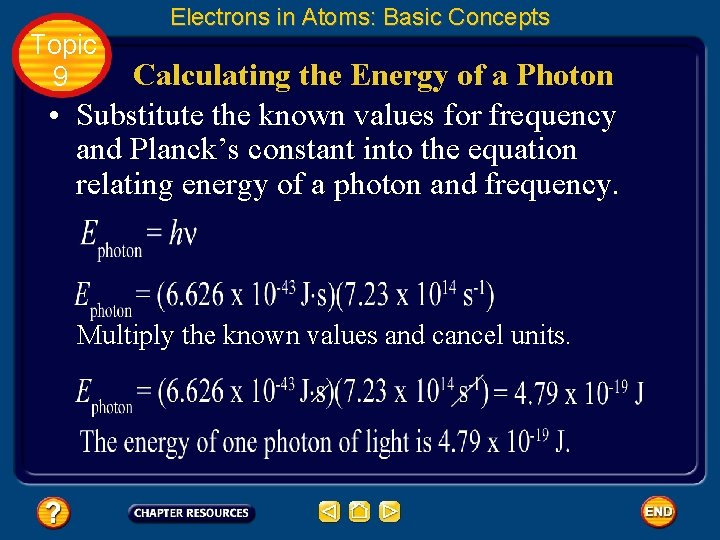Topic 9 Electrons in Atoms: Basic Concepts Calculating the Energy of a Photon • Substitute the known values for frequency and Planck’s constant into the equation relating energy of a photon and frequency. Multiply the known values and cancel units.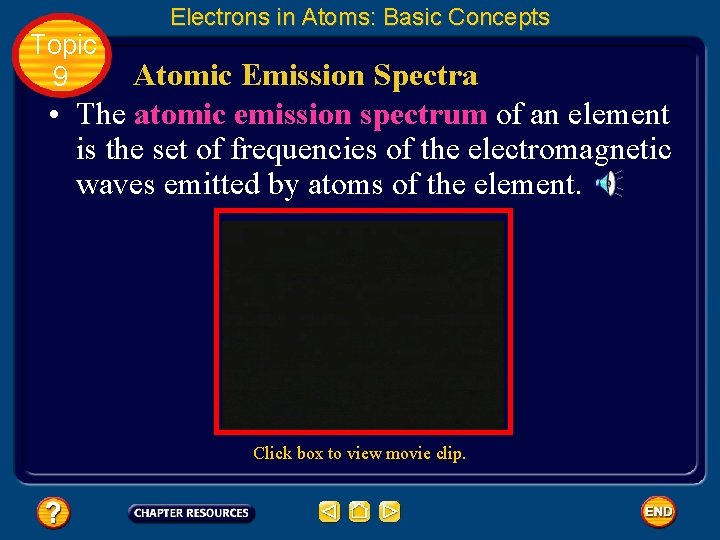Topic 9 Electrons in Atoms: Basic Concepts Atomic Emission Spectra • The atomic emission spectrum of an element is the set of frequencies of the electromagnetic waves emitted by atoms of the element. Click box to view movie clip.Topic 9 Electrons in Atoms: Basic Concepts Atomic Emission Spectra • Neon’s atomic emission spectrum consists of several individual lines of color, not a continuous range of colors as seen in the visible spectrum. • Each element’s atomic emission spectrum is unique and can be used to determine if that element is part of an unknown compound.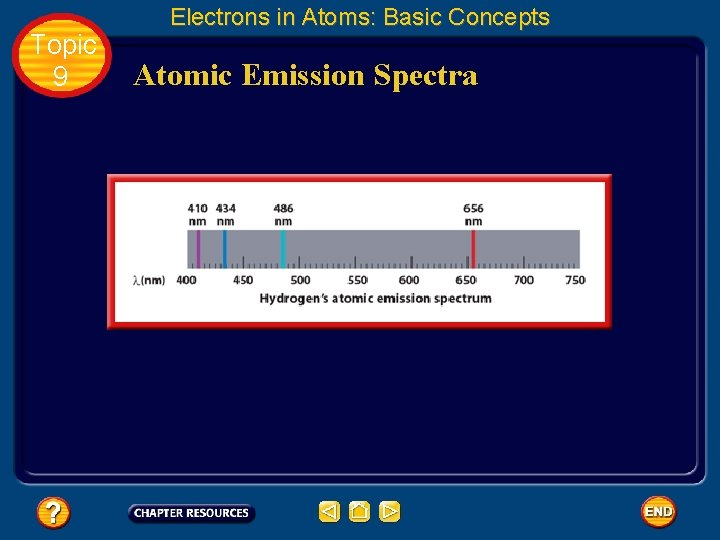Topic 9 Electrons in Atoms: Basic Concepts Atomic Emission Spectra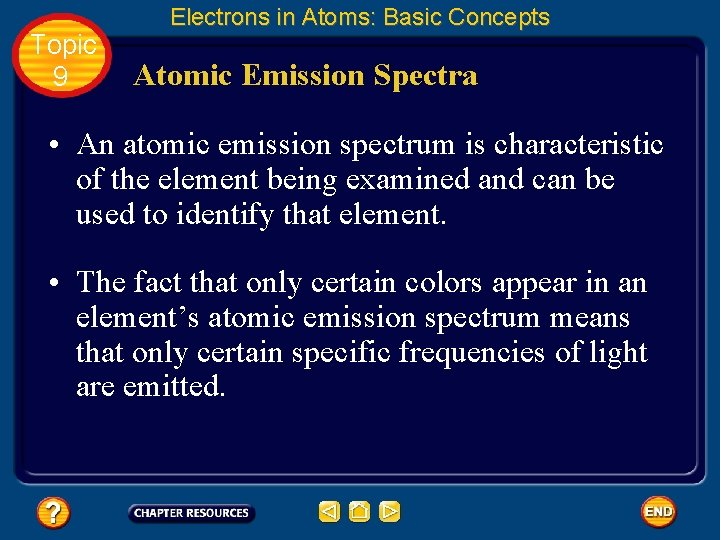Topic 9 Electrons in Atoms: Basic Concepts Atomic Emission Spectra • An atomic emission spectrum is characteristic of the element being examined and can be used to identify that element. • The fact that only certain colors appear in an element’s atomic emission spectrum means that only certain specific frequencies of light are emitted.Topic 9 Electrons in Atoms: Basic Concepts Atomic Emission Spectra • And because those emitted frequencies of light are related to energy by the formula Ephoton = hn, it can be concluded that only photons having certain specific energies are emitted.Topic 9 Electrons in Atoms: Basic Concepts Atomic Emission Spectra • Scientists found atomic emission spectra puzzling because they had expected to observe the emission of a continuous series of colors and energies as excited electrons lost energy and spiraled toward the nucleus.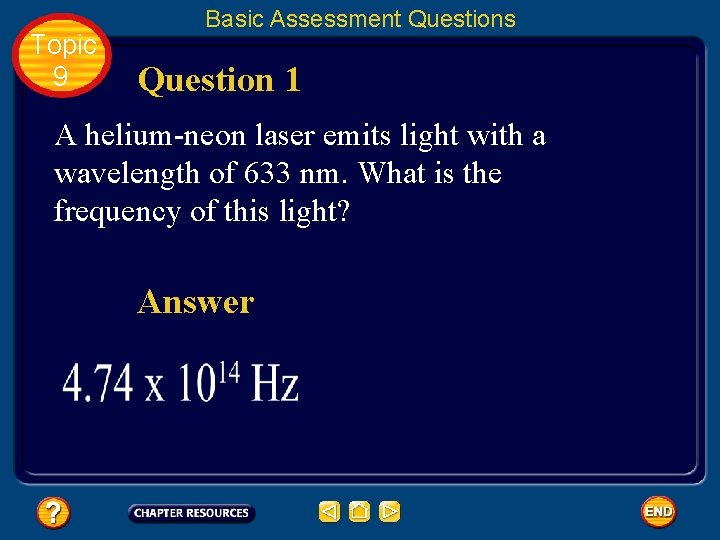Topic 9 Basic Assessment Questions Question 1 A helium-neon laser emits light with a wavelength of 633 nm. What is the frequency of this light? AnswerTopic 9 Basic Assessment Questions Question 2 What is the wavelength of X rays having a frequency of 4. 80 x 1017 Hz? AnswerTopic 9 Basic Assessment Questions Question 3 An FM radio station broadcasts at a frequency of 98. 5 MHz. What is the wavelength of the station’s broadcast signal? AnswerTopic 9 Basic Assessment Questions Question 4 Calculate the energy of a gamma ray photon whose frequency is 5. 02 x 1020 Hz. Answer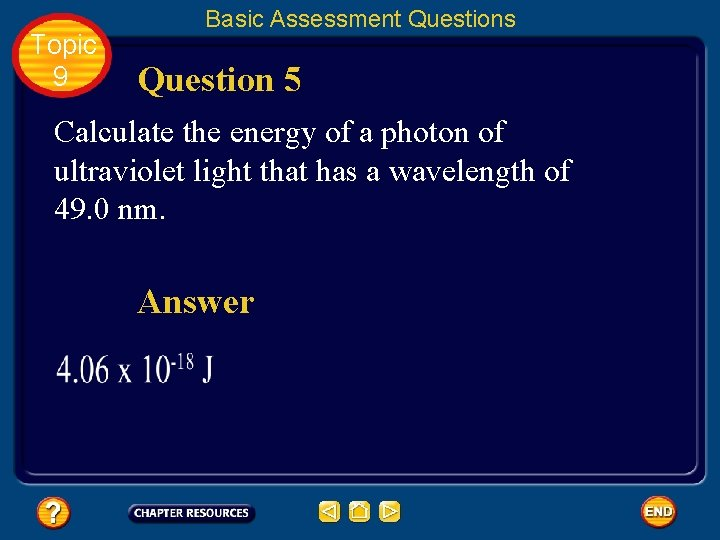Topic 9 Basic Assessment Questions Question 5 Calculate the energy of a photon of ultraviolet light that has a wavelength of 49. 0 nm. Answer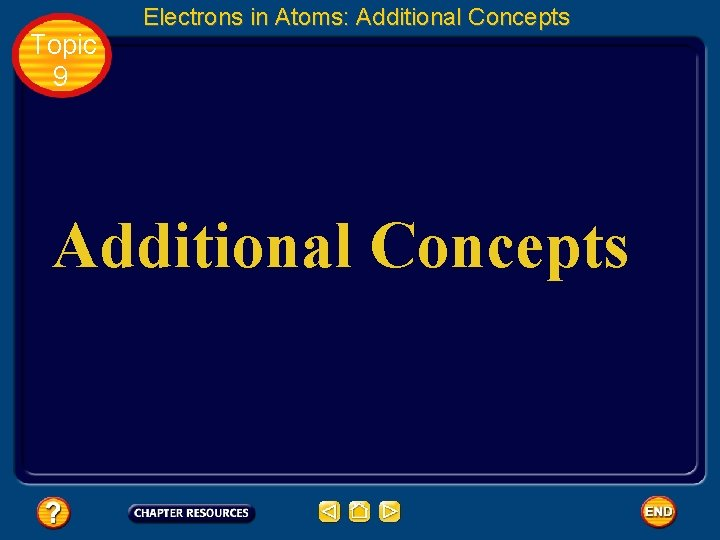Topic 9 Electrons in Atoms: Additional Concepts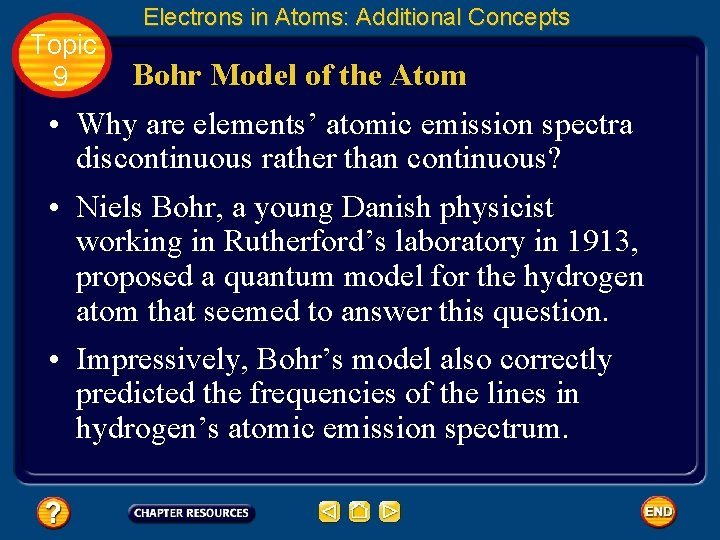Topic 9 Electrons in Atoms: Additional Concepts Bohr Model of the Atom • Why are elements’ atomic emission spectra discontinuous rather than continuous? • Niels Bohr, a young Danish physicist working in Rutherford’s laboratory in 1913, proposed a quantum model for the hydrogen atom that seemed to answer this question. • Impressively, Bohr’s model also correctly predicted the frequencies of the lines in hydrogen’s atomic emission spectrum.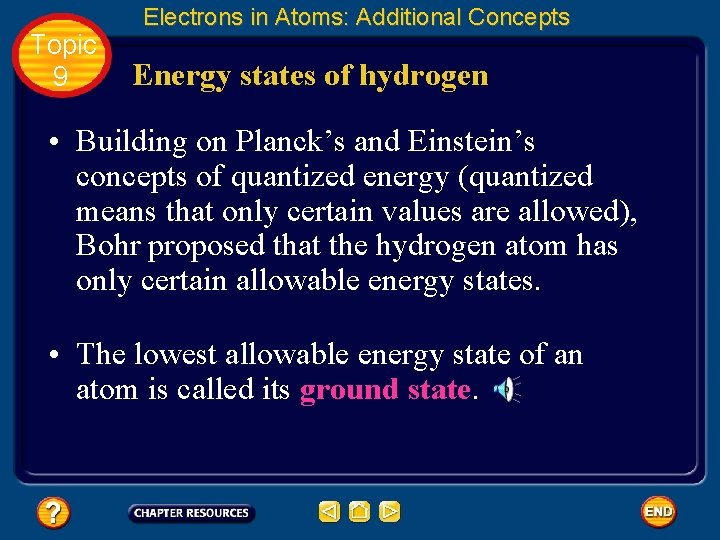Topic 9 Electrons in Atoms: Additional Concepts Energy states of hydrogen • Building on Planck’s and Einstein’s concepts of quantized energy (quantized means that only certain values are allowed), Bohr proposed that the hydrogen atom has only certain allowable energy states. • The lowest allowable energy state of an atom is called its ground state.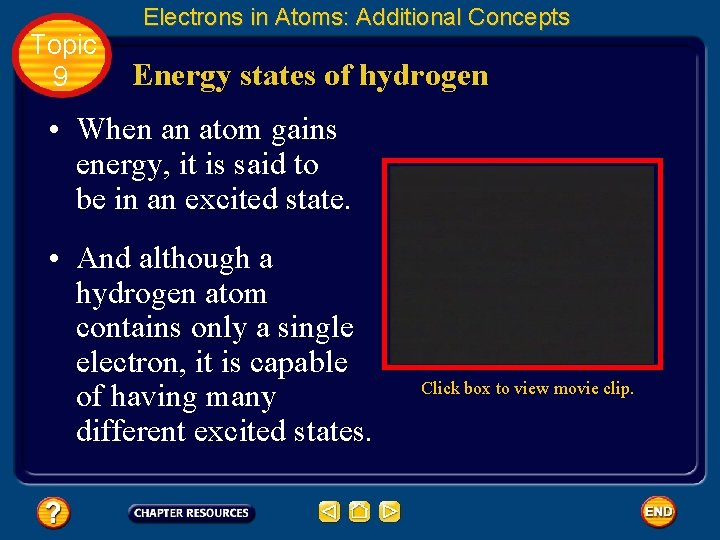Topic 9 Electrons in Atoms: Additional Concepts Energy states of hydrogen • When an atom gains energy, it is said to be in an excited state. • And although a hydrogen atom contains only a single electron, it is capable of having many different excited states. Click box to view movie clip.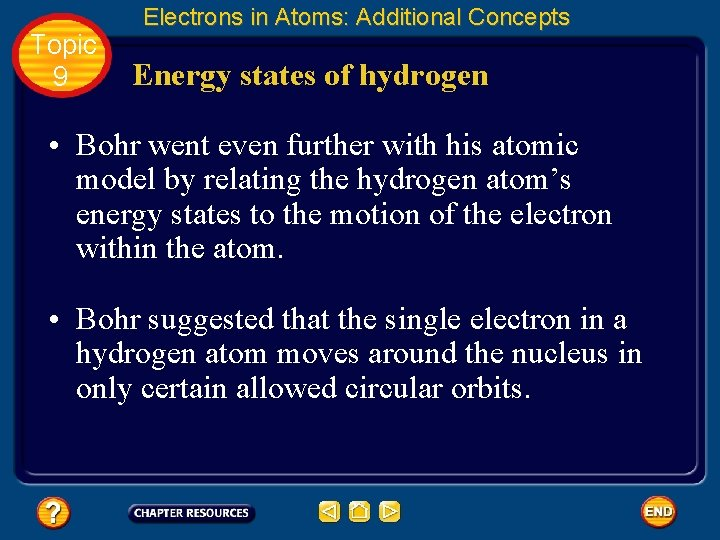Topic 9 Electrons in Atoms: Additional Concepts Energy states of hydrogen • Bohr went even further with his atomic model by relating the hydrogen atom’s energy states to the motion of the electron within the atom. • Bohr suggested that the single electron in a hydrogen atom moves around the nucleus in only certain allowed circular orbits.Topic 9 Electrons in Atoms: Additional Concepts Energy states of hydrogen • The smaller the electron’s orbit, the lower the atom’s energy state, or energy level. • Conversely, the larger the electron’s orbit, the higher the atom’s energy state, or energy level.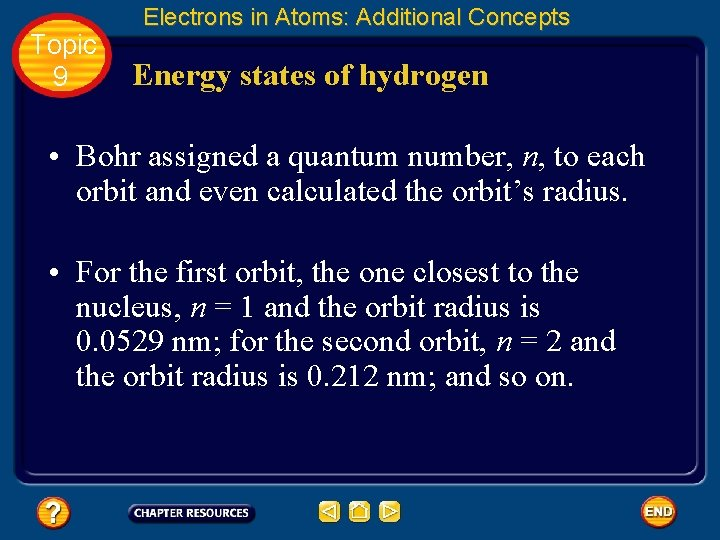Topic 9 Electrons in Atoms: Additional Concepts Energy states of hydrogen • Bohr assigned a quantum number, n, to each orbit and even calculated the orbit’s radius. • For the first orbit, the one closest to the nucleus, n = 1 and the orbit radius is 0. 0529 nm; for the second orbit, n = 2 and the orbit radius is 0. 212 nm; and so on.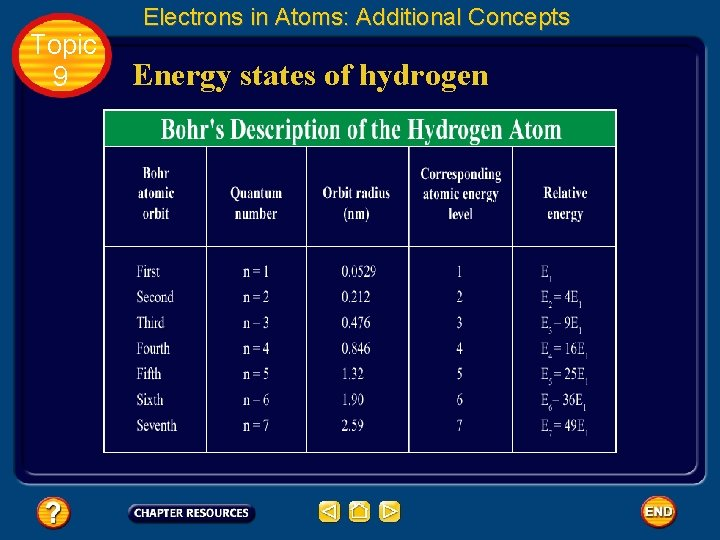Topic 9 Electrons in Atoms: Additional Concepts Energy states of hydrogenTopic 9 Electrons in Atoms: Additional Concepts An explanation of hydrogen’s line spectrum • Bohr suggested that the hydrogen atom is in the ground state, also called the first energy level, when the electron is in the n = 1 orbit.Topic 9 Electrons in Atoms: Additional Concepts An explanation of hydrogen’s line spectrum • In the ground state, the atom does not radiate energy when energy is added from an outside source, the electron moves to a higher-energy orbit such as the n = 2 orbit shown.Topic 9 Electrons in Atoms: Additional Concepts An explanation of hydrogen’s line spectrum • Such an electron transition raises the atom to an excited state. • When the atom is in an excited state, the electron can drop from the higher-energy orbit to a lower-energy orbit. • As a result of this transition, the atom emits a photon corresponding to the difference between the energy levels associated with the two orbits.Topic 9 Electrons in Atoms: Additional Concepts An explanation of hydrogen’s line spectrum • The four electron transitions that account for visible lines in hydrogen’s atomic emission spectrum are shown.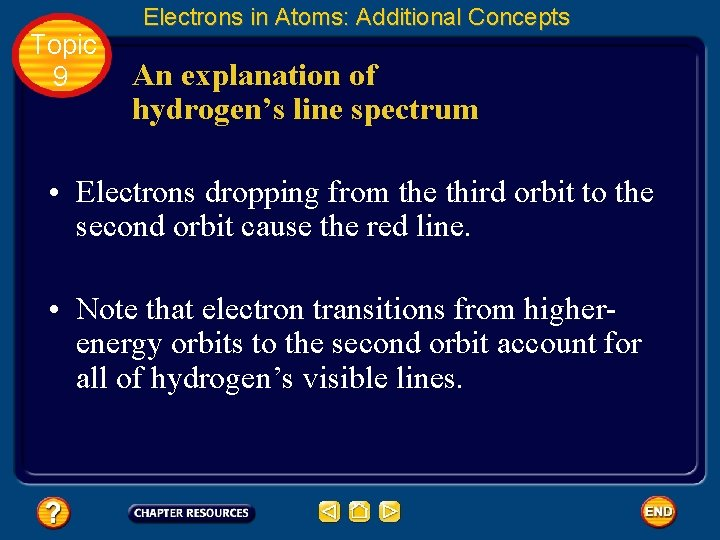Topic 9 Electrons in Atoms: Additional Concepts An explanation of hydrogen’s line spectrum • Electrons dropping from the third orbit to the second orbit cause the red line. • Note that electron transitions from higherenergy orbits to the second orbit account for all of hydrogen’s visible lines.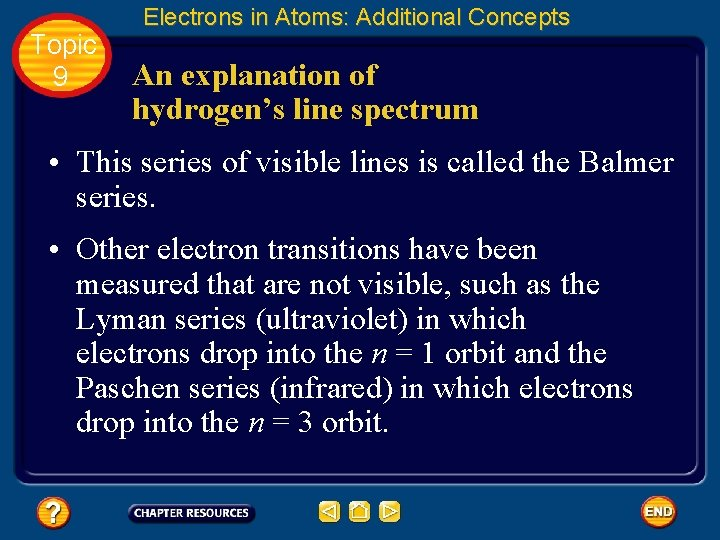Topic 9 Electrons in Atoms: Additional Concepts An explanation of hydrogen’s line spectrum • This series of visible lines is called the Balmer series. • Other electron transitions have been measured that are not visible, such as the Lyman series (ultraviolet) in which electrons drop into the n = 1 orbit and the Paschen series (infrared) in which electrons drop into the n = 3 orbit.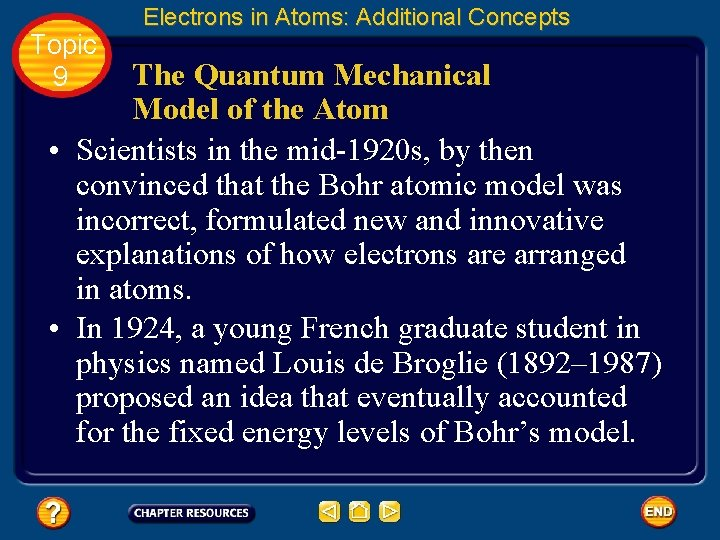Topic 9 Electrons in Atoms: Additional Concepts The Quantum Mechanical Model of the Atom • Scientists in the mid-1920 s, by then convinced that the Bohr atomic model was incorrect, formulated new and innovative explanations of how electrons are arranged in atoms. • In 1924, a young French graduate student in physics named Louis de Broglie (1892– 1987) proposed an idea that eventually accounted for the fixed energy levels of Bohr’s model.Topic 9 Electrons in Atoms: Additional Concepts Electrons as waves • De Broglie had been thinking that Bohr’s quantized electron orbits had characteristics similar to those of waves.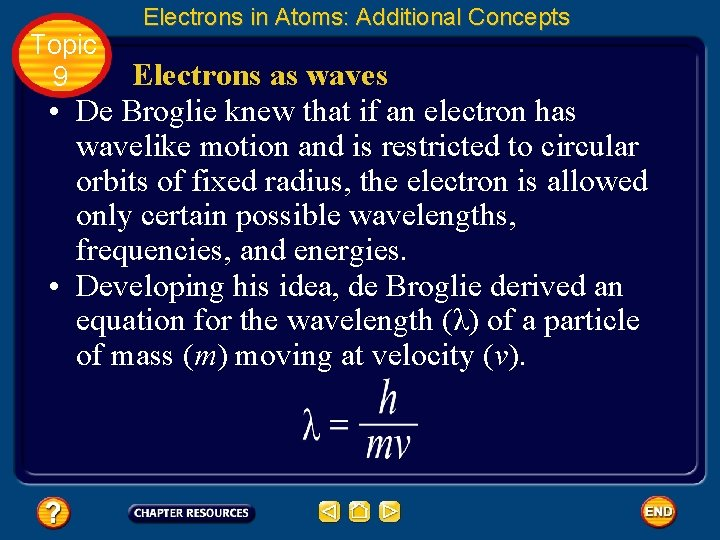Topic 9 Electrons in Atoms: Additional Concepts Electrons as waves • De Broglie knew that if an electron has wavelike motion and is restricted to circular orbits of fixed radius, the electron is allowed only certain possible wavelengths, frequencies, and energies. • Developing his idea, de Broglie derived an equation for the wavelength (λ) of a particle of mass (m) moving at velocity (ν).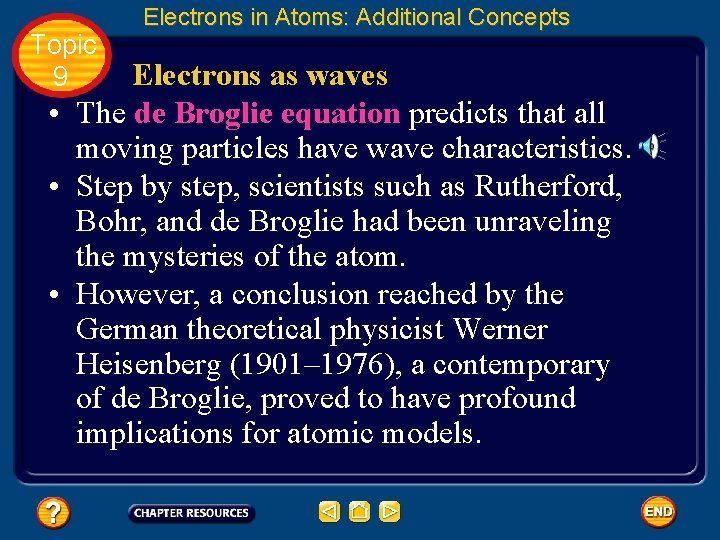Topic 9 Electrons in Atoms: Additional Concepts Electrons as waves • The de Broglie equation predicts that all moving particles have wave characteristics. • Step by step, scientists such as Rutherford, Bohr, and de Broglie had been unraveling the mysteries of the atom. • However, a conclusion reached by the German theoretical physicist Werner Heisenberg (1901– 1976), a contemporary of de Broglie, proved to have profound implications for atomic models.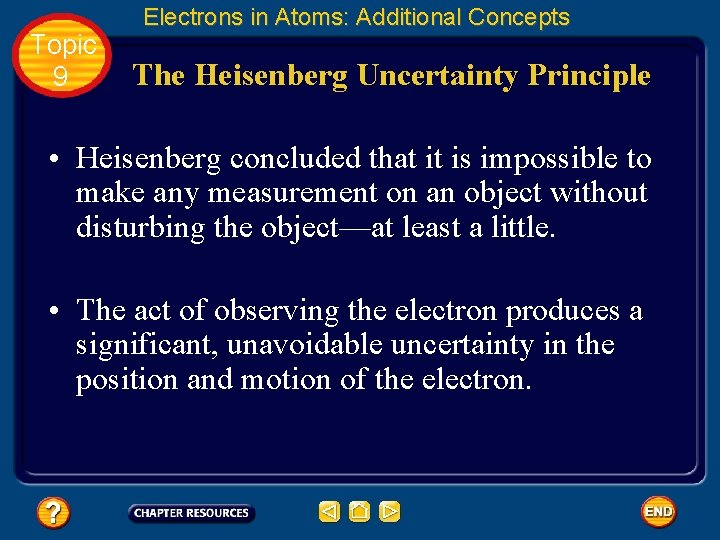Topic 9 Electrons in Atoms: Additional Concepts The Heisenberg Uncertainty Principle • Heisenberg concluded that it is impossible to make any measurement on an object without disturbing the object—at least a little. • The act of observing the electron produces a significant, unavoidable uncertainty in the position and motion of the electron.Topic 9 Electrons in Atoms: Additional Concepts The Heisenberg Uncertainty Principle • Heisenberg’s analysis of interactions such as those between photons and electrons led him to historic conclusion. • The Heisenberg uncertainty principle states that it is fundamentally impossible to know precisely both the velocity and position of a particle at the same time.Topic 9 Electrons in Atoms: Additional Concepts The Schrödinger wave equation • In 1926, Austrian physicist Erwin Schrödinger (1887– 1961) furthered the wave-particle theory proposed by de Broglie. • Schrödinger derived an equation that treated the hydrogen atom’s electron as a wave. • Remarkably, Schrödinger’s new model for the hydrogen atom seemed to apply equally well to atoms of other elements—an area in which Bohr’s model failed.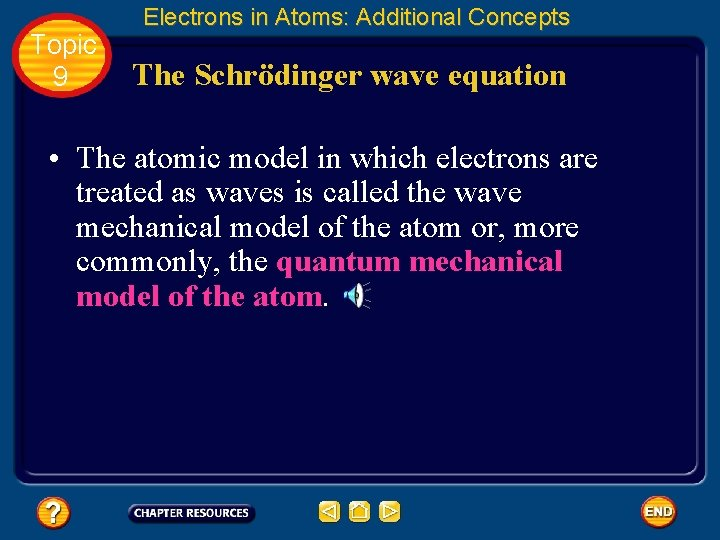Topic 9 Electrons in Atoms: Additional Concepts The Schrödinger wave equation • The atomic model in which electrons are treated as waves is called the wave mechanical model of the atom or, more commonly, the quantum mechanical model of the atom.Topic 9 Electrons in Atoms: Additional Concepts The Schrödinger wave equation • Like Bohr’s model, the quantum mechanical model limits an electron’s energy to certain values. • However, unlike Bohr’s model, the quantum mechanical model makes no attempt to describe the electron’s path around the nucleus.Topic 9 Electrons in Atoms: Additional Concepts The Schrödinger wave equation • A three-dimensional region around the nucleus called an atomic orbital describes the electron’s probable location. • You can picture an atomic orbital as a fuzzy cloud in which the density of the cloud at a given point is proportional to the probability of finding the electron at that point.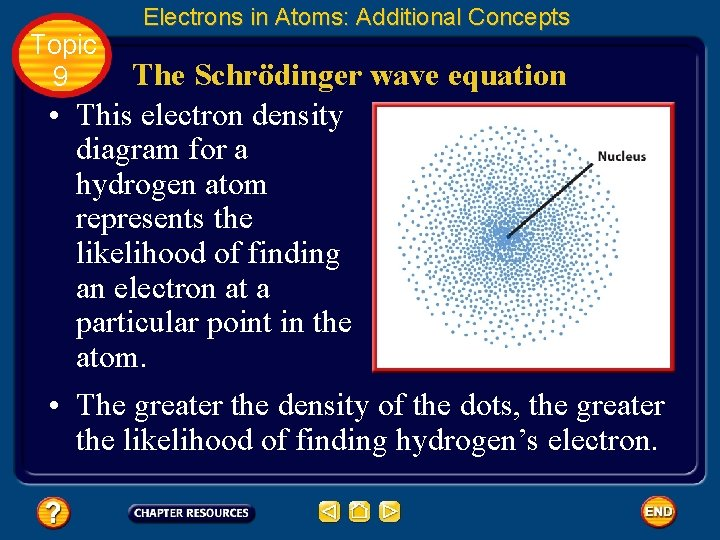Topic 9 Electrons in Atoms: Additional Concepts The Schrödinger wave equation • This electron density diagram for a hydrogen atom represents the likelihood of finding an electron at a particular point in the atom. • The greater the density of the dots, the greater the likelihood of finding hydrogen’s electron.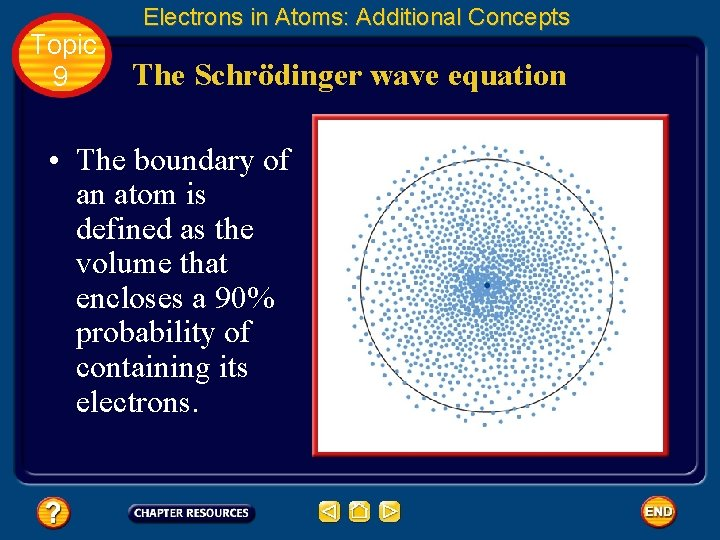Topic 9 Electrons in Atoms: Additional Concepts The Schrödinger wave equation • The boundary of an atom is defined as the volume that encloses a 90% probability of containing its electrons.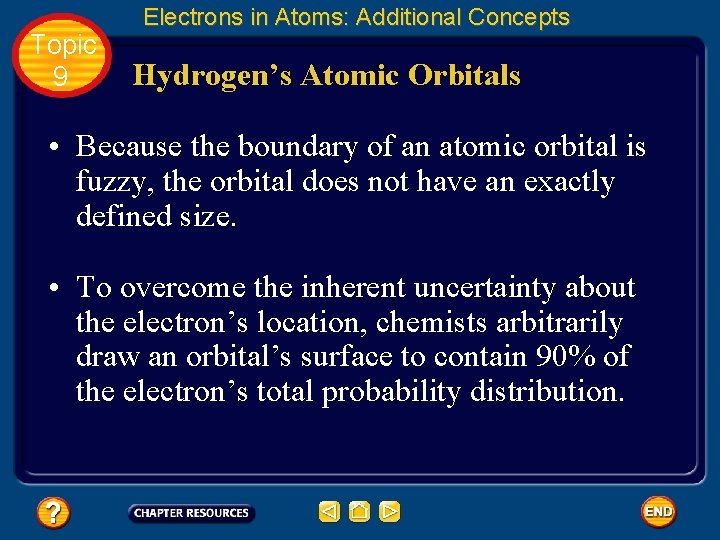Topic 9 Electrons in Atoms: Additional Concepts Hydrogen’s Atomic Orbitals • Because the boundary of an atomic orbital is fuzzy, the orbital does not have an exactly defined size. • To overcome the inherent uncertainty about the electron’s location, chemists arbitrarily draw an orbital’s surface to contain 90% of the electron’s total probability distribution.Topic 9 Electrons in Atoms: Additional Concepts Hydrogen’s Atomic Orbitals • In other words, the electron spends 90% of the time within the volume defined by the surface, and 10% of the time somewhere outside the surface.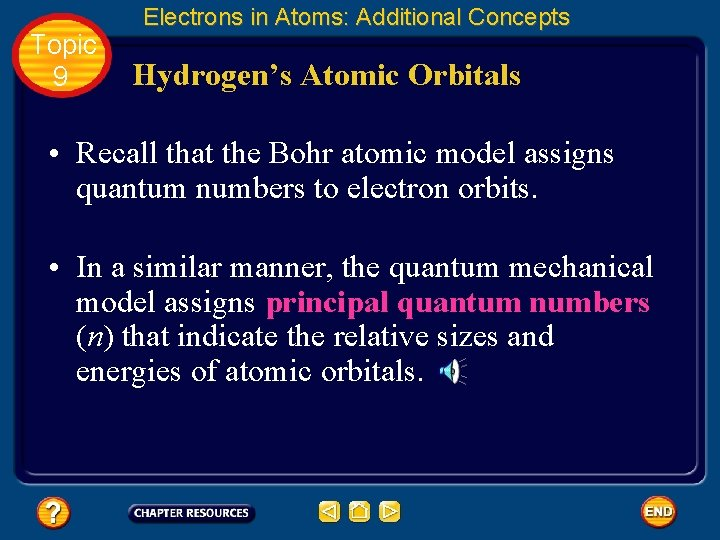Topic 9 Electrons in Atoms: Additional Concepts Hydrogen’s Atomic Orbitals • Recall that the Bohr atomic model assigns quantum numbers to electron orbits. • In a similar manner, the quantum mechanical model assigns principal quantum numbers (n) that indicate the relative sizes and energies of atomic orbitals.Topic 9 Electrons in Atoms: Additional Concepts Hydrogen’s Atomic Orbitals • That is, as n increases, the orbital becomes larger, the electron spends more time farther from the nucleus, and the atom’s energy level increases. • Therefore, n specifies the atom’s major energy levels, called principal energy levels.Topic 9 Electrons in Atoms: Additional Concepts Hydrogen’s Atomic Orbitals • An atom’s lowest principal energy level is assigned a principal quantum number of one. • When the hydrogen atom’s single electron occupies an orbital with n = 1, the atom is in its ground state. • Up to seven energy levels have been detected for the hydrogen atom, giving n values ranging from 1 to 7.Topic 9 Electrons in Atoms: Additional Concepts Hydrogen’s Atomic Orbitals • Principal energy levels contain energy sublevels. • Principal energy level 1 consists of a single sublevel, principal energy level 2 consists of two sublevels, principal energy level 3 consists of three sublevels, and so on.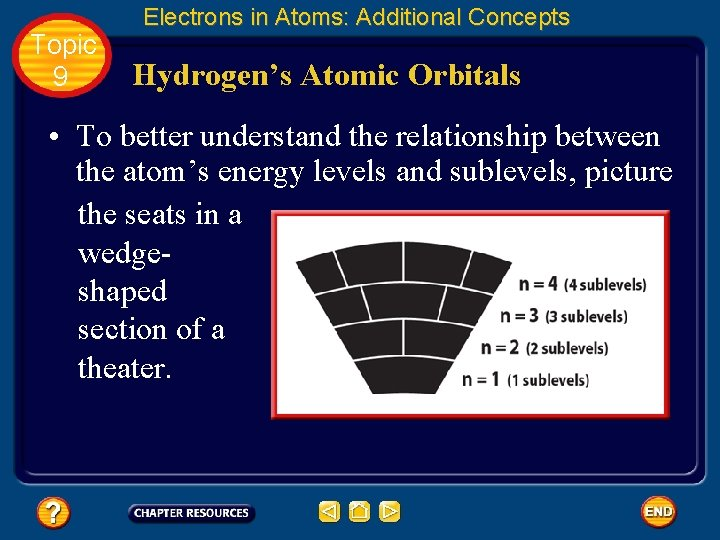Topic 9 Electrons in Atoms: Additional Concepts Hydrogen’s Atomic Orbitals • To better understand the relationship between the atom’s energy levels and sublevels, picture the seats in a wedgeshaped section of a theater.Topic 9 Electrons in Atoms: Additional Concepts Hydrogen’s Atomic Orbitals • As you move away from the stage, the rows become higher and contain more seats. • Similarly, the number of energy sublevels in a principal energy level increases as n increases.Topic 9 Electrons in Atoms: Additional Concepts Hydrogen’s Atomic Orbitals • Sublevels are labeled s, p, d, or f according to the shapes of the atom’s orbitals. • All s orbitals are spherical and all p orbitals are dumbbell shaped; however, not all d or f orbitals have the same shape.Topic 9 Electrons in Atoms: Additional Concepts Hydrogen’s Atomic Orbitals • Each orbital may contain at most two electrons. • The single sublevel in principal energy level 1 consists of a spherical orbital called the 1 s orbital.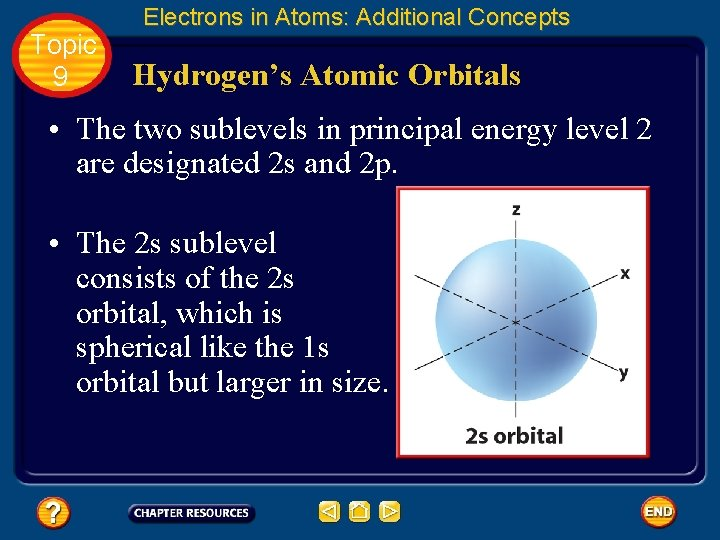Topic 9 Electrons in Atoms: Additional Concepts Hydrogen’s Atomic Orbitals • The two sublevels in principal energy level 2 are designated 2 s and 2 p. • The 2 s sublevel consists of the 2 s orbital, which is spherical like the 1 s orbital but larger in size.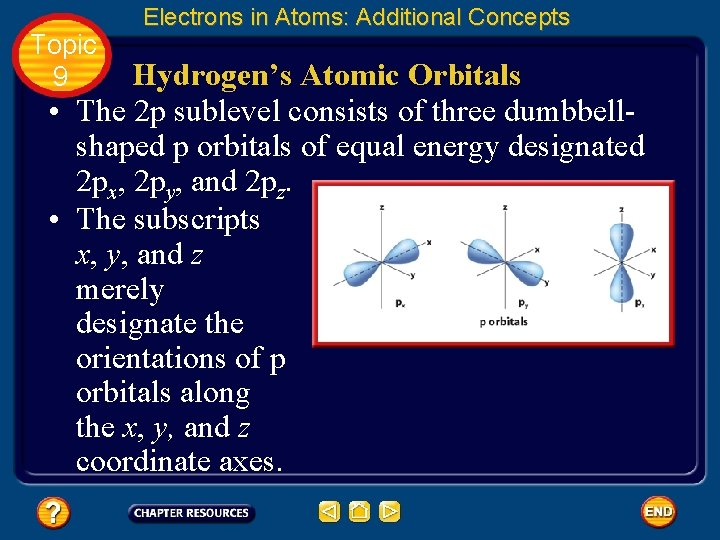Topic 9 Electrons in Atoms: Additional Concepts Hydrogen’s Atomic Orbitals • The 2 p sublevel consists of three dumbbellshaped p orbitals of equal energy designated 2 px, 2 py, and 2 pz. • The subscripts x, y, and z merely designate the orientations of p orbitals along the x, y, and z coordinate axes.Topic 9 • • Electrons in Atoms: Additional Concepts Hydrogen’s Atomic Orbitals Principal energy level 3 consists of three sublevels designated 3 s, 3 p, and 3 d. Each d sublevel consists of five orbitals of equal energy. Four d orbitals have identical shapes but different orientations. However, the fifth, dz 2 orbital is shaped and oriented differently from the other four.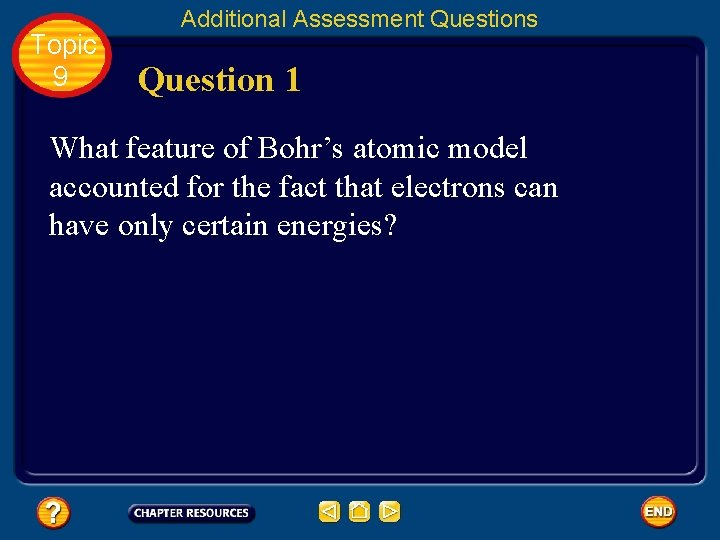Topic 9 Additional Assessment Questions Question 1 What feature of Bohr’s atomic model accounted for the fact that electrons can have only certain energies?Topic 9 Additional Assessment Questions Answer Bohr’s model showed that electrons move in circular orbits around the nucleus. The electrons have energies associated with those orbits.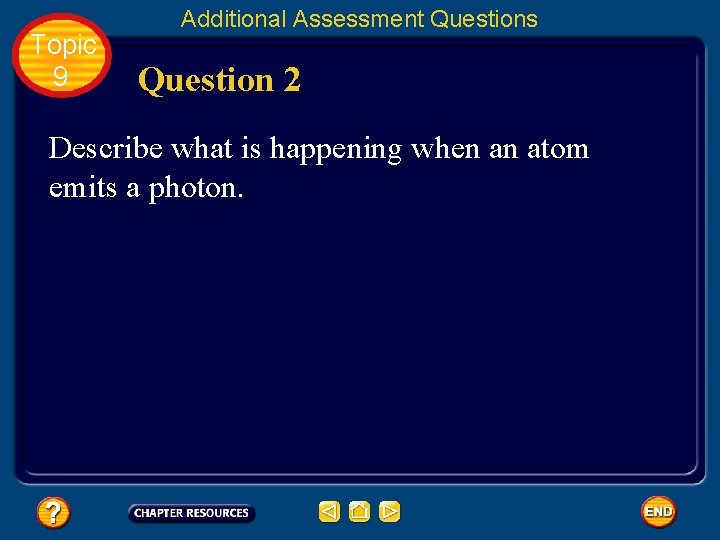Topic 9 Additional Assessment Questions Question 2 Describe what is happening when an atom emits a photon.Topic 9 Additional Assessment Questions Answer The electron is moving from an orbital of higher energy to an orbital of lower energy.Topic 9 Additional Assessment Questions Question 3 How many electrons can the second principal energy level hold? How many electrons can the third principal energy level hold? Explain the difference in these numbers of electrons.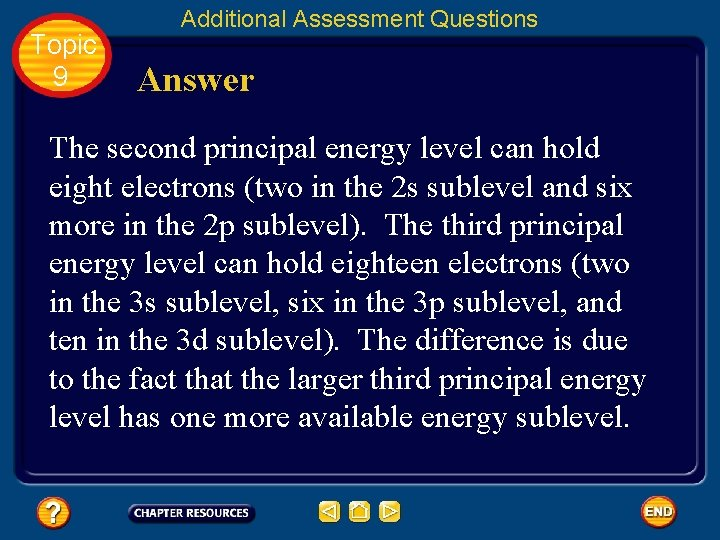Topic 9 Additional Assessment Questions Answer The second principal energy level can hold eight electrons (two in the 2 s sublevel and six more in the 2 p sublevel). The third principal energy level can hold eighteen electrons (two in the 3 s sublevel, six in the 3 p sublevel, and ten in the 3 d sublevel). The difference is due to the fact that the larger third principal energy level has one more available energy sublevel.Help To advance to the next item or next page click on any of the following keys: mouse, space bar, enter, down or forward arrow. Click on this icon to return to the table of contents Click on this icon to return to the previous slide Click on this icon to move to the next slide Click on this icon to open the resources file. Click on this icon to go to the end of the presentation.End of Topic Summary File